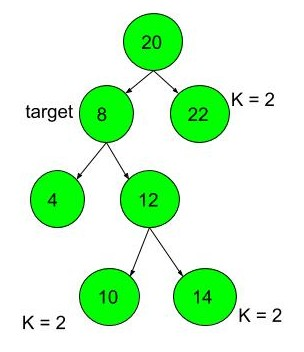Nodes at given distance in binary tree
##### Submissions: 8827   Accuracy: 39.45%   Difficulty: Hard   Marks: 8

Given a binary tree, a target node in the binary tree, and an integer value k, print all the nodes that are at distance k from the given target node. No parent pointers are available.

Input:
The first line of input contains an integer T denoting the number of test cases. Then T test cases follow. Each test case contains an integer n denoting the number of edges. Then the following line contains the edges as given in the example. The next line contains the target node. The last line of input contains the the value of distance k.

Output:
Print all the space separated nodes that are at distance k from the given target node.

Constraints:
1 <= T <= 50
1 <= no of edges <= 100
1<= data of node <= 100
1 <= target <= 100
1 <= k <= 10

Example:
Input:

2
6
20 8 L 20 22 R 8 4 L 8 12 R 12 10 L 12 14 R
8
2
5
20 7 L 20 24 R 7 4 L 7 3 R 3 1 L
7
2

Output:
10 14 22
1 24

Explanation:
Testcase 1:we can observe that target node is 8,  and nodes which are at k distant from this target node are 10, 14 and 22.

#### ** For More Input/Output Examples Use 'Expected Output' option **

Contributor: Ayush Govil
Author: Ayush Govil 1

If you have purchased any course from GeeksforGeeks then please ask your doubt on course discussion forum. You will get quick replies from GFG Moderators there.